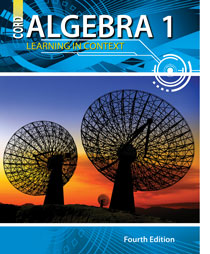# Algebra 1 - 4th Edition

## Chapter 9: Exponential and Radical Functions and Operations

Some links are repeated for use with more than one lesson.

### 9.1 Functions Involving Square and Cube Roots

http://www.explorelearning.com/index.cfm?method=cResource.dspDetail&ResourceID=107
This site contains a gizmo that explores functions involving square roots.

This is the first of a sequence of pages that present strategies for solving equations with square roots, cube roots, and fourth roots.

This site presents and provides practice for solving equations with square roots.

http://www.analyzemath.com/Equations/equations_cube_root.html
This site is a general guide on solving equations containing cube roots.

### 9.2 Irrational Numbers and Radicals

http://www.mathsisfun.com/algebra/exponent-fractional.html
This page shows the relation between radicals and fractional exponents, and some of the rules for working with them.

http://www.mathsisfun.com/irrational-numbers.html

http://www.mathsisfun.com/algebra/rationalize-denominator.html

http://www.regentsprep.org/Regents/math/algtrig/ATO3/indexATO3.htm
This site provides a presentation and practice on radicals and rationalizing simple and complicated denominators that contain radicals.

http://www.regentsprep.org/Regents/math/algtrig/ATE10/indexATE10.htm
This site presents and provides practice for solving equations with square roots.

### 9.4 The Pythagorean Theorem

http://www.cut-the-knot.org/pythagoras/index.shtml
This page defines the Pythagorean Theorem and has several proofs explaining it.

http://www.mathsisfun.com/pythagoras.html
This site explains the Pythagorean Theorem and has pictures showing the sides of a triangle.

### 9.5 Exponential Growth and Decay

http://www.regentsprep.org/regents/math/algebra/AE7/ExpDecayL.htm
This page explains exponential growth and decay with notes, tables, and graphs.

http://hotmath.com/help/gt/genericalg1/section_9_6.html

### 9.6 Choosing an Appropriate Function Model

http://www.mathsisfun.com/algebra/mathematical-models.html
This site helps you think about writing equations that model a real world scenario.

### 9.7 Geometric Sequences

http://www.freemathhelp.com/Lessons/Algebra_2_Sequences_and_Series_BB.htm
This site contains a video tutorial with notes on arithmetic and geometric sequences and series.

http://www.mathsisfun.com/algebra/sequences-series.html
This page explores the rules and notations used with sequences and series, and includes practice problems.

http://www.regentsprep.org/Regents/math/algtrig/ATP3/indexATP3.htm
This site discusses and provides practice with recursive formulas that generate sequences.

http://www.purplemath.com/modules/series3.htm
This site links to several pages discussing sequences, but this page examines arithmetic and geometric sequences in particular.